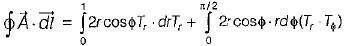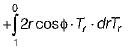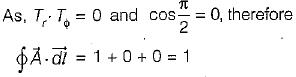Courses

# Magnetostatics - 2

## 20 Questions MCQ Test Topicwise Question Bank for Electrical Engineering | Magnetostatics - 2

Description
This mock test of Magnetostatics - 2 for Electrical Engineering (EE) helps you for every Electrical Engineering (EE) entrance exam. This contains 20 Multiple Choice Questions for Electrical Engineering (EE) Magnetostatics - 2 (mcq) to study with solutions a complete question bank. The solved questions answers in this Magnetostatics - 2 quiz give you a good mix of easy questions and tough questions. Electrical Engineering (EE) students definitely take this Magnetostatics - 2 exercise for a better result in the exam. You can find other Magnetostatics - 2 extra questions, long questions & short questions for Electrical Engineering (EE) on EduRev as well by searching above.
QUESTION: 1

### A circular conductor of 1 cm radius has an internal magnetic field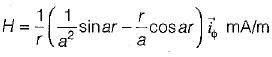where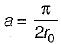, r0 is the radius of the conductor and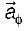is the unit vector. The total current in the conductor is given by

Solution:

By Ampere's law,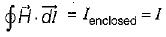Hence, current enclosed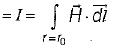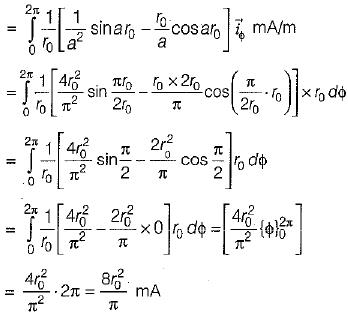Given, r0 = 1 cm = 0.01 m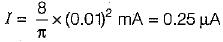QUESTION: 2

### What is the value of mutual inductance between an infinitely long straight conductor along the y-axis and a rectangular single turn coil situated in x-y plane with its corners located at point (a, 0), (a + d, 0), (a, h) and (a + d, h) as shown in figure below?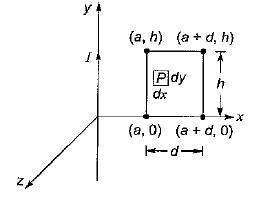Solution:

The mutual inductance is given by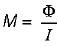Flux density at any point P in the x-y plane is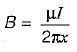and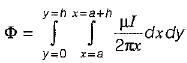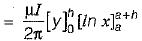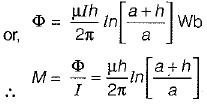QUESTION: 3

### Assertion (A): The force acting between two parallel wires carrying currents is directly proportional to the individual currents and inversely proportional to the square of the distance between them, Reason (R): The force is repulsive if the two currents I1 and I2 are in the same directions and attractive if in opposite directions.

Solution:

The force acting between the two parallel wires carrying currents I2 and I2 respectively is given by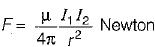Thus,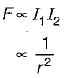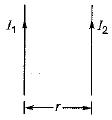Hence, assertion is a correct statement.
Reason is a false statement because F will be attractive if the two currents I2 and I2 will be in the same directions and repulsive if in opposite directions.

QUESTION: 4

The energy stored in a magnetic field is given by

Solution:
QUESTION: 5

Lorentz force law is

Solution:
QUESTION: 6

The equation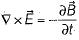is the generalization of

Solution:
QUESTION: 7

The unit of relative permeability is

Solution:

Relative permeability,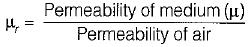μr is a dimensionless quantity.

QUESTION: 8

Consider the volume current density distribution in cylindrical co-ordinates as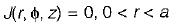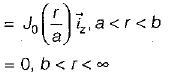Where a and b are inner and outer radii of the cylinder as shown in figure below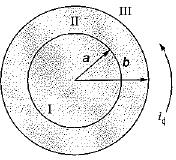Now, consider the value of magnetic field intensity in various regions I, II and III respectively obtained as: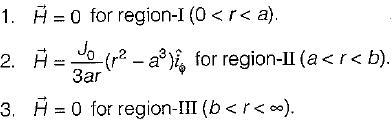Which of the above found values of H are correct for the respective regions?

Solution:
• For region-I (0 < r < a), J (r, ϕ, z) = 0
Using Ampere’s law, we have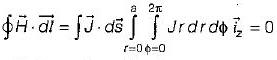or , H·2πr = 0 or H = 0
• For region - II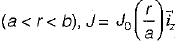Again,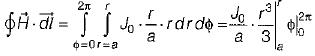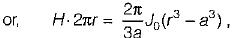or,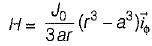• For region - III  (b < r < ∞), J = 0
Again,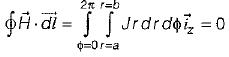QUESTION: 9

Assertion (A): It is not possible to have isolated magnetic charges.
Reason (R): The magnetic flux lines always close upon themselves.

Solution: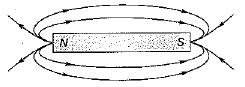Since, magnetic flux lines always close upon themselves therefore it is not possible to have isolated magnetic charges (or poles).

QUESTION: 10

Match List-l with List-lI and select the correct answer using the codes given below the fists:
List-l
A. Gauss’s law for magnetostatic fields
B. Gauss’s law for electrostatic fields
C. Conservativeness of electrostatic fields
D. Ampere's law
List-lI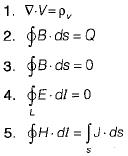Codes:
A B C D
(a) 5 4 1 2
(b) 3 1 4 5
(c) 3 4 1 5
(d) 5 1 4 2

Solution:
QUESTION: 11

The magnetic vector potential is given by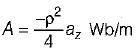The total magnetic flux crossing the surface ϕ = π/2 , 1 ≤ ρ ≤ 2 m , 0 ≤ z ≤ 5 m is

Solution:

The total magnetic flux crossing the surface
ϕ = π/2 , 1 ≤ ρ ≤ 2 m , 0 ≤ z ≤ 5 m is given as: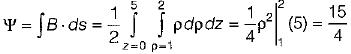= 3.75 Wb

QUESTION: 12

Which of the following is not a source of magnetostatic fields?

Solution:

An accelerated charge will produce both time varying electric and magnetic field called electromagnetic field.

QUESTION: 13

Consider the following statements associated with the characteristics of static magnetic field:
1. It is solenoidal.
2. Magnetic flux lines are not closed in certain cases.
3. The total number of flux lines entering a given region is equal to the total number of flux lines leaving the region.
4. It is conservative.
Which of the above statements is/are not correct?

Solution:
• Static magnetic field is non-conservative unlike static electric field. Hence, statement-4 is not correct.
• Magnetic flux lines are always closed. Hence, statement-2 is not correct.
• Statement 1 and 3 are correct.
QUESTION: 14

What are the units for the product of two values whose respective units are Henrys and Amperes?

Solution:

The Henry, the unit for mutual inductance is equivalent to V-sec/Ampere.
So, H x Ampere = Volt-sec = V-s

QUESTION: 15

What are the possible dimensions for a rectangular coil that has a magnetic flux of 9.5 webers when in a magnetic field of strength 19 Tesla at an angle of 60° from the perpendicular to the plane of the coil?

Solution:

Magnetic flux φB = BA cosθ
or,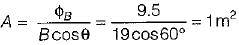Hence, the area of the rectangular coil should be 1 m2. Only option (d) is matching the answer i.e. 250 cm x 40 cm
= 10000 cm2 = 1 m2.

QUESTION: 16

What is the change in magnetic fiux in a coil of area 5 m2 as its orientation relative to the perpendicular of a uniform 3.0 T magnetic field changes from 45° to 90°?

Solution:

Flux ϕ = B A cosθ
At θ = 45°,   ϕ1= 3 x 5 x cos 45° = 11 Wb
At θ = 90°,    ϕ2 = 3 x 5 x cos 90° = 0 Wb
∴ Change in flux = ϕ2 - ϕ1 = -11 Wb

QUESTION: 17

If a vector field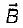is solenoidal, which of these is true?

Solution: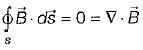(Non-existence of monopole)

QUESTION: 18

If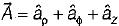, the value of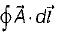around the closed circular quadrant shown in the given figure is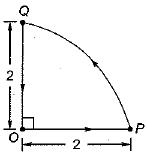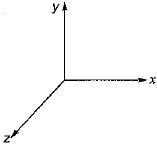Solution: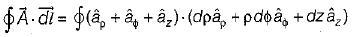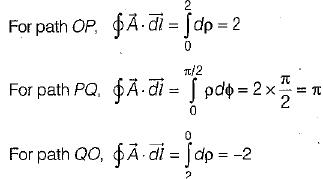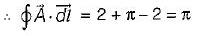QUESTION: 19

The Maxwell’s equation,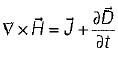is based on

Solution:
QUESTION: 20

Given a vector field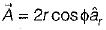in cylindrical coordinates. For the contour as shown below,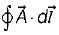is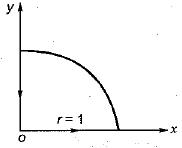Solution: